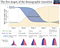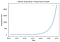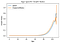# Using Python in Demography: Part 1

Happy New Year to everyone! Given the start of the New Year (and the beginning of my preparation for my Finals), I thought it would be apt to start a mini-series on… Demography! With all the confusion that’s been going on in the world in the last year, maybe it would be good to try to understand people better. Which, Demography (defined as “the study of human populations in relation to the changes brought about by the interplay of births, deaths, and migration” (Pressat, 1985)) prompt fulfills. This will likely be a 4 part series, looking at 2 demographic measures per part. Each part will look at the theoretical concepts behind the measure and then explore using Python to easily calculate it. So… Let’s dive right in!

# Theory

Three key theoretical points about population growth rates before we dive into calculations.With permission from ourworldindata.org

# Growth Equation

In order to calculate the change in a population, we use the following equation:

K(t+n) =K(t) + B(t,t+n) − D(t,t+n) + I(t,t+n) − E(t,t+n)

where t is the current time and n is how many years later we are projecting forward. B is births, D is deaths, I is immigration, and E is emigration. K(t) is the original size of the population so this is intuitive enough

Since population growth is a multiplicative process, we can find K(1) by taking K(0) (the previous time period) and multiply that by (1 + B/K — D/K). Here, we take the ratios of births over population minus deaths over population. In Demography, we call this A. So, simplified:

where K(T) is the population at any time period and K(0) is the current time period. A assumes a constant birth and death rate. Let’s plot this out shall we?Graph (by author)

We see that population growth is exponential. What can we do with this? Well, in order to get R (the growth rate), we need to take ln(A). This gives us the following equation:

ln(K(t)) = ln(K(0)) + R*T

This is the final equation to find the growth rate and this can be used in many useful ways. For instance, we could find the doubling time — the time it takes for a population to double, assuming a constant growth rate.

# Theory

The theory behind this measure was much simpler. Essentially, we need to calculate death rates since these are essential rates in looking at population growth. There are 2 types of death rates — crude and standardized. Crude rates are so called because:

In order to compare populations, one has to thus use age-specific rates and standardize the populations. To standardize a population, we assume that population A has the same age structure as population and coerce it to be so.

# Calculations

Calculating the age specific death rates really isn’t that difficult. The first method was to basically replicate what one would do in excel and create all the individual columns for the rates before summing it up. This could potentially be useful if one wanted to graph out some of the data. I show this on a sample dataset comparing Japan and England/Wales.

`Japan Standardized Death Rate: 0.00741 England/Wales Standardized Death Rate: 0.01090`Graph (by author)

Alternatively, one could combine everything into one big messy chunk of code like so. Both would return the same results except that one would be slightly more efficient.

`Japan Standardized Death Rate: 0.00741 England/Wales Standardized Death Rate: 0.01090`

That’s the end of part 1 where we looked at simple measures of population growth and standardized death rates! In part 2, we’ll take a look at mortality rates as well as period vs cohort measures! Thanks for reading :)

Originally published at zachlim98.github.io/me.

Hi! I’m learning to explore data and think about personal finance (not always in that order)